# Silicon Nanowire Polarization Rotator

## Tools Used: FullWAVE, FemSIM and MOST

Si photonic devices are very attractive in modern communication technologies due to their high performance and low-cost fabrication, and Si devices have been developed to fulfill numerous different aspects of new communication technologies, such as optical interconnects requiring ultra-high data-transmission capacity.

A polarization rotator is one of most essential devices used to manipulate polarization. Polarization rotators can be either active or passive. This RSoft products application note illustrates an ultra-small passive polarization rotator that is simple to fabricate and operate. This rotator is based on a Si nanowire with an asymmetrical cross-section as shown in Fig.1a.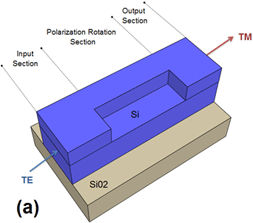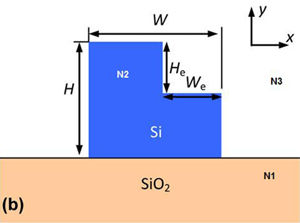Figure 1: The Si nanowire polarization rotator used in this tutorial: a) oblique view schematic and b) cross-sectional view.

The schematic shown in Fig. 1a illustrates the polarization rotator studied in this application note. It includes three parts: a polarization input section (set as TE in this case), a polarization rotation section, and a polarization output section (TM in this case). For the polarization rotator, the first two modes are sufficiently hybrid that their optical axes are rotated 45° with respect to the fixed coordinates of the device (x,y in Fig.1b), respectively. When linearly polarized TE light is launched into this longitudinally invariant waveguide, the two modes are excited with almost equal amplitude. At each half beat-length, full 90°-polarization rotation is achieved and TM output is achieved. The half beat-length Lπ is determined from the propagation constants β1, β2 of the two first modes as Lπ = π/( β1 - β2).

To achieve a shorter half-beat length Lπ, a high-index contrast waveguide is preferred. To give a detailed analysis, we will use a FullWAVE simulation to compute the Polarization Conversion Efficiency (PCE), which is defined as: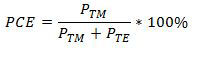## Calculating the TE/TM Modes

Here we use the built-in BPM-based iterative mode solver included in FullWAVE to calculate the TE/TM modes in the polarization rotator. The more rigorous FEM-based mode solver FemSIM can also be used to get these results.

TE Mode in the Input/Output Sections: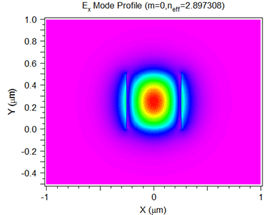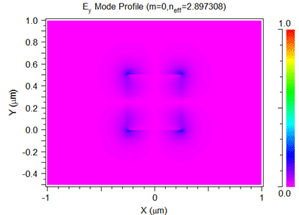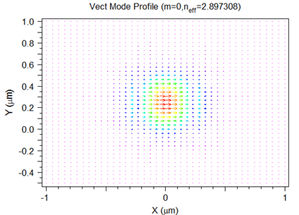Figure 2: The computed TE mode for the input/output sections: a) Ex component, b) Ey component, and c) a vector plot of E.

TM Mode in the Output Section: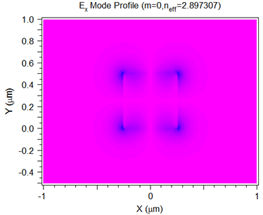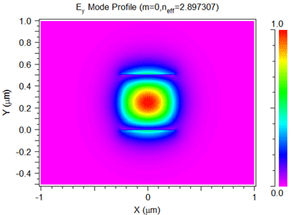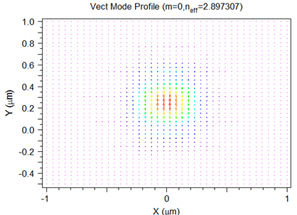Figure 3: The computed TM mode for the output section: a) Ex component, b) Ey component, and c) a vector plot of E.

## TE/TM Modes in the Polarization-Rotation Section: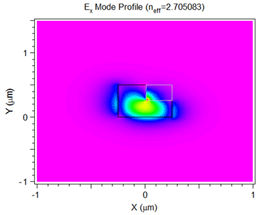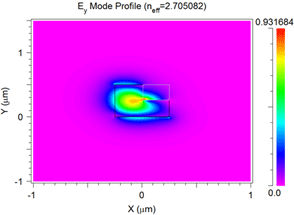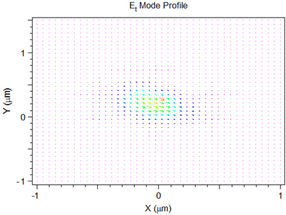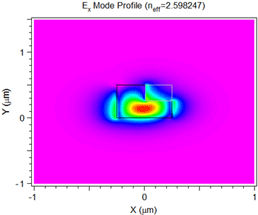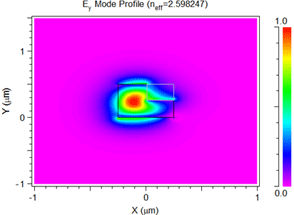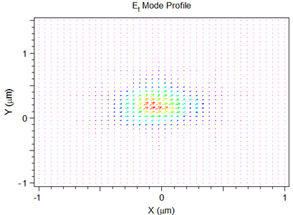Figure 4: The TE (top row) and TM (bottom row) modes calculated in the rotator section. These modes were calculated using the FEM-based FemSIM mode solver.

Note that the fields in the Fig 4 vector plots are tilted at approximately +/- 45 degrees, and that both modes are perpendicular to each other. When linearly polarized light is launched into this waveguide, both of these modes will be excited and, at each half beat-length, a full 90 degree polarization rotation will be achieved.

## Simulating the Polarization Rotator

As shown in Fig. 5, simulating the polarization rotator structure (see Fig. 1) in FullWAVE clearly shows the polarization rotation from TE to TM.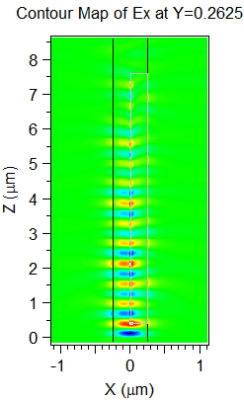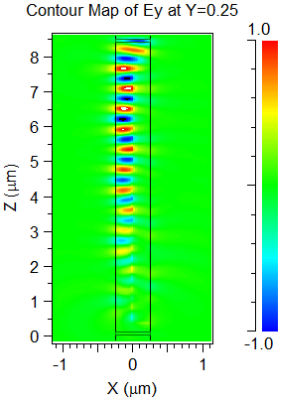Fig 6: The MOST scan results in two formats: a) standard output using plotting options, and b) contour plot.

## Calculating the Polarization Conversion Efficiency (PCE)

The PCE, defined at the start of this application note, measures the power percentage transferred to the orthogonally polarized mode from the polarized input field. The PCE depends on the etching width We and depth He. We can use RSoft's Multi-Variable Optimization and Scanning Tool, MOST, to scan over We and He and automatically calculate the PCE.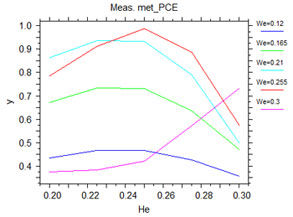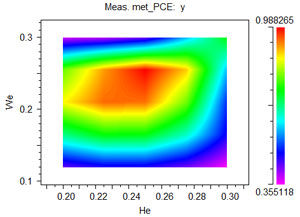Fig 6: The MOST scan results in two formats: a) standard output using plotting options, and b) contour plot.

## References

 Z.Wang et al: "Ultrasmall Si-nanowire-based polarization rotator" J. Opt. Soc. Am. B Vol.25, No.5, May 2008.

 J.Z.Huang et al:"Realization of a Compact and Single-Mode Optical Passive Polarization Converter" IEEE Photonics Technology Letters, Vol.12, No.3, March 2000